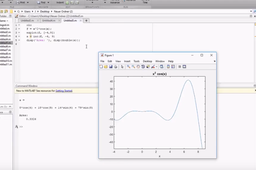# [VIDEO] Matlab Tutorial: Solving integral equations Part 1How to achieve a solution for integral equations with the aid of Matlab?
This tutorial shows how to do gather a solution!